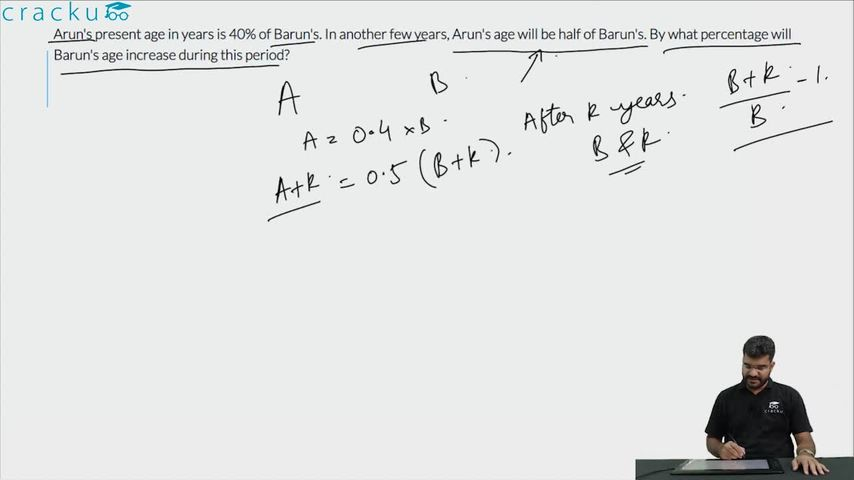Question 67

# Arun's present age in years is 40% of Barun's. In another few years, Arun's age will be half of Barun's. By what percentage will Barun's age increase during this period?

Solution

Let Arun's current age be A. Hence, Barun's current age is 2.5A
Let Arun's age be half of Barun's age after X years.
Therefore, 2*(X+A) = 2.5A + X
Or, X = 0.5A
Hence, Barun's age increased by 0.5A/2.5A = 20%

### View Video Solution• All Quant CAT Formulas and shortcuts PDF
• 30+ CAT previous papers with solutions PDF